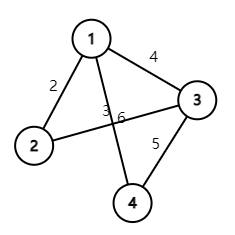0%

# 一、简析对偶理论

König定理：一张二分图的最大匹配数等于最小点覆盖数。

Definition 1$$A$$$$n\times m$$矩阵、$$x$$$$n\times1$$向量、$$c$$$$1\times n$$向量、$$y$$$$1\times m$$向量、$$b$$$$m\times 1$$向量，原问题为： \begin{align*} \max\text{ }&z=cx\\ s.t.\text{ }&Ax\leq b,\\ &x\geq 0.\end{align*}\tag{1}\label{1} 则其对偶问题为： \begin{align*} \min\text{ }&w=yb\\ s.t.\text{ }&yA\geq c,\\ &y\geq 0.\end{align*}\tag{2}\label{2} 接着来介绍对偶问题中与本文内容比较相关的几个性质：

Theorem 1(弱对偶性)$$\hat{x},\hat{y}$$分别是原问题$$\eqref{1}$$与其对偶问题$$\eqref{2}$$的可行解，则$$c\hat{x}\leq yb$$

• Proof 由(1)，有$$A\hat{x}\leq b$$，且$$\hat{y}\geq 0$$，则$$\hat{y}A\hat{x}\leq\hat{y}b$$
由(2)，有$$yA\geq c$$，且$$\hat{x}\geq0$$，则$$\hat{y}A^{T}\hat{x}\geq cx$$，从而$$c\hat{x}\leq yb$$$$\Box$$

Theorem 2(最优性)$$\hat{x},\hat{y}$$分别是原问题$$\eqref{1}$$与其对偶问题$$\eqref{2}$$的可行解，且$$c\hat{x}=\hat{y}b$$，则它们分别是两个问题的最优解。

• Proof$$x^*$$$$\eqref{1}$$的最优解，则$$\hat{y}b=c\hat{x}\leq cx^*$$，又由弱对偶性，$$cx^*\leq\hat{y}b$$，则$$cx^*=\hat{y}b=c\hat{x}$$$$\Box$$

Theorem 3(强对偶性) 原问题与对偶问题其中一个有最优解，则另一个也有最优解，且最优解的值相等。

# 二、网络流中的对偶问题$$\eqref{3}$$式的表述，对这个网络，有$$P=\{\{(1,2),(2,3),(3,4)\},\{(1,3),(3,4)\},\{(1,4)\}\}$$，最大流问题可表示为： \begin{align*} &\max\sum_{p\in P}f_p\\ s.t.\text{ }&\begin{pmatrix} 1&0&0\\ 0&1&0\\ 0&0&1\\ 1&0&0\\ 1&1&0 \end{pmatrix}\begin{pmatrix}f_{p_1}\\f_{p_2}\\f_{p_3}\end{pmatrix} \leq \begin{pmatrix} 2\\ 4\\ 3\\ 6\\ 5 \end{pmatrix},\\ &f_{p_1},f_{p_2},f_{p_3}\geq0.\end{align*} 其对偶问题为： \begin{align*} &\min (y_1,y_2,y_3,y_4,y_5)\begin{pmatrix} 2\\4\\3\\6\\5\end{pmatrix}\\ s.t.\text{ }&(y_1,y_2,y_3,y_4,y_5)\begin{pmatrix} 1&0&0\\ 0&1&0\\ 0&0&1\\ 1&0&0\\ 1&1&0 \end{pmatrix}\geq\begin{pmatrix} 1\\1\\1\end{pmatrix},\\ &y_1,y_2,y_3,y_4,y_5\geq0.\end{align*} 通过这个例子很清晰的就能看出两者之间的联系。

• Proof$$\hat{y}$$是一个最优解，显然$$\forall e\in E,0\leq\hat{y}(e)\leq 1$$。若$$\exists e'\in E,0<\hat{y}(e')<1$$，设$$P'=\{p\in P|e'\in p\}$$
任取$$P'$$中的一条路径$$p$$，一定还存在除了$$e'$$之外的边满足$$\hat{y}$$值大于$$0$$，每条路径中都取出这样的一条边，构成一个边集$$E'$$
假设$$\sum_{e\in E'}c(e)>c(e')$$，设$$e_0\in\text{argmax}_{e\in E'}(c(e))$$，考虑如下解： $y^*(e)\coloneqq\begin{cases} \hat{y}(e)-\hat{y}(e_0)&\text{if e\in E',}\\ \hat{y}(e)+\hat{y}(e_0)&\text{if e=e',}\\ \hat{y}(e)&\text{else.} \end{cases}$ 则有
\begin{align*} \sum_{e\in E}c(e)y^*(e)&=\sum_{e\in E\backslash({E'}\cup\{e'\}}c(e)\hat{y}(e)+\sum_{e\in E'}c(e)(\hat{y}(e)-\hat{y}(e_0))+c(e')(\hat{y}(e')+\hat{y}(e_0))\\ &=\sum_{e\in E}c(e)\hat{y}(e)+(c(e')-\sum_{e\in E'}c(e))\hat{y}(e_0)\\ &<\sum_{e\in E}c(e)\hat{y}(e). \end{align*} 这与$$\hat{y}$$为最优解矛盾，因此$$\sum_{e\in E'}c(e)\leq c(e')$$。同理可得$$\sum_{e\in E'}c(e)\geq c(e')$$，从而$$\sum_{e\in E'}c(e)=c(e')$$
那么考虑如下解： $\overline{y}\coloneqq\begin{cases} \hat{y}(e)+\hat{y}(e')&\text{if e\in E',}\\ 0&\text{if e=e',}\\ \hat{y}(e)&\text{else.} \end{cases}$ 很显然$$\sum_{e\in E}c(e)\bar{y}(e)=\sum_{e\in E}c(e)\hat{y}(e)$$，即$$\bar{y}$$也是最优解。
每进行一次上述的转化，满足$$y$$值在$$(0,1)$$上的边的数量至少减少一条，因此进行有限次转化，即可使得所有边的$$y$$值都大于等于$$1$$，又由所有$$y$$值一定小于等于$$1$$，因此这个解的取值就在$$\{0,1\}$$上了，所以一定存在一个取值在$$\{0,1\}$$上的最优解。 $$\Box$$

# 三、二分图匹配中的最大最小

Lemma 1 任取$$G$$中的一个点覆盖$$V'=A'\cup B',A'\subset A,B'\subset B$$，则$$[\{s\}\cup A',\{t\}\cup B']$$$$G'$$的一个切割，且包含了$$G'$$的所有切割，即$$G$$的点覆盖与$$G'$$的切割构成一个双射。